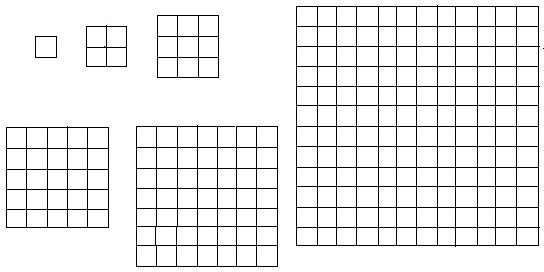# Building Square Patios and Their Roots: 8th Grade Math Lesson on Perfect Squares

In this lesson, you are a contractor who specializes in building patios in the shape of perfect squares and during the building of the patios in the shape of perfect squares you discover the square roots of the perfect squares.

Lesson Objective: Lesson is aligned to the Common Core State Standards for Mathematics – 8.EE.2 Expressions and Equations – Use square root and cube root symbols to represent solutions to equations of the form x2 = p and x3 = p, where p is a positive rational number. Evaluate square roots of small perfect squares and cube roots of small perfect cubes. Know that  is irrational.

Materials Required: dot paper, graph paper, square tiles (In the activities, students may replace the dot paper for graph paper or square tiles)

## Lesson ProcedureIn this lesson, you are a contractor who specializes in building patios in the shape of perfect squares with square tiles. The numbers of tiles used for each patio are perfect squares. You discover the lengths of the sides of the patios are the square roots of the perfect squares.

Activity 1

1. On dot paper, draw the smallest square patio. The length of each of the four sides of the patio should measure 1 unit.
2. To find the area of the patio, count the number of small squares inside the patio. This is the number of tiles you need to build this patio.
3. How many tiles are needed to build this patio?
• Answer: 1

Activity 2

1. On dot paper, draw the next smallest square patio. The length of each of the four sides of the patio should measure 2 units.
2. To find the area of the patio, count the number of small squares inside the patio. This is the number of tiles you need to build this patio.
3. How many tiles are needed to build this patio?
• Answer: 4

Activity 3

1. On dot paper, draw the next smallest square patio. The length of each of the four sides of the patio should measure 3 units.
2. To find the area of the patio, count the number of small squares inside the patio. This is the number of tiles you need to build this patio.
3. How many tiles are needed to build this patio?
• Answer: 9

Discuss with students that the area of a square patio is found by multiplying the length of the side by itself (s x s) or squaring the side (s2). Discuss with students when writing the area in exponential form (s2), the base number is the length of the side and the exponent is 2.

Discuss with students that a square root is the inverse operation of squaring a number. You may relate this to subtraction is the inverse operation of addition and division is the inverse operation of multiplication.

Download & Have Students Complete This Table

Individual or Group Work

After this lesson, students be able to square a number between 1 and 15 and be able to find the square root of perfect squares between 1 and 225.

## This post is part of the series: Working with Radicals and Integer Exponents

This series of lessons is suitable for students in Grade 8. It covers working with positive and negative integer exponents and radicals. Students will be presented with real world scenarios and practice problems.